# DAV Class 6 Maths Chapter 2 Worksheet 5 Solutions

The DAV Class 6 Maths Book Solutions Pdf and DAV Class 6 Maths Chapter 2 Worksheet 5 Solutions of Factors and Multiples offer comprehensive answers to textbook questions.

## DAV Class 6 Maths Ch 2 WS 5 Solutions

Question 1.
Find the HCF of the following numbers by the factor method:

(a) 7, 18
Solution:
7, 18
Factors of 7 = , 7
Factors of 18 = 1. 2, 3, 6, 9, 18
Common factor = 1
HCF = 1

(b) 12, 30, 54
Solution:
12, 30, 54
Factors of 12 = 1, 2, 3, 4, 6, 12
Factors of 30 = 1, , 3, 5, 6, 10, 15, 3
Factors of 54 = 1, 2,3, 6,9, 18, 27, 5
Common factor = 1, 2, 3, 6
∴ Highest common factor (HCF) = 6

(c) 70, 14, 35
Solution:
70, 14, 35
Factors of 70 = 1, 2, 5, 7, 10, 14, 35, 70
Factors of 14 = 1, 2, 7, 14
Factors of 35 = 1 5, 7, 35
Common factors = 1 × 7 = 7
∴ HCF = 7Question 2.
Find the HCF of the following numbers by prime factorisation method:

(a) 76, 28
Solution:
76, 28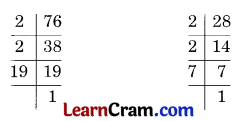76 = 2 × 2 × 19
28 = 2 × 2 × 7
Prime factors of 76 = 2 × 2 × 19
Prime factors of 28 = 2 × 2 × 7
Common factors are 2 and 2.
∴ HCF = 2 × 2 = 4

(b) 24, 16, 36
Solution:24 = 2 × 2 × 2 × 3 → Prime factors of 24
16 = 2 × 2 × 2 × 2 → Prime factors of 16
36 = 2 × 2 × 3 × 3 → Prime factors of 36
Common factors are 2 and 2.
∴ HCF = 2 × 2 = 4

(c) 38, 64, 82
Solution:38 = 2 × 19 → Prime factors of 38
64 = 2 × 2 × 2 × 2 × 2 × 2 → Prime factors of 64
32 = 2 × 2 × 2 × 2 × 2 → Prime factors of 32
Common factors is 2.
∴ HCF = 2Question 3.
Find HCF of the following numbers by continued division method.

(a) 345, 506
Solution:
Here, 506 > 345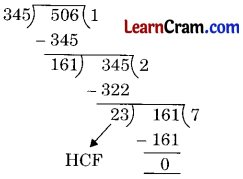Hence, HCF of 345 and 506 is 23

(b) 144, 384, 120
Solution:
Here, 384 > 144 > 120The HCF of 384 and 144 = 48
Now HCF of 48 and 144 is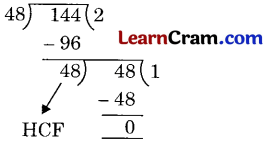Hence, the required HCF = 48(c) 287, 533
Solution:
Here, 533 > 287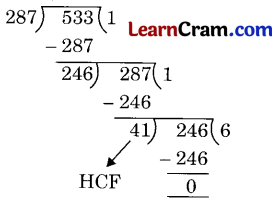Hence, the required HCF = 41

(d) 208, 494, 949
Solution:
Here, 949 > 494 > 208Now we have to calculate the HCF of 13 and 208Hence, HCF of 208, 494, 949 = 13.(e) 1212, 6868, 1111
Solution:
Here, 6868 > 1212 > 1111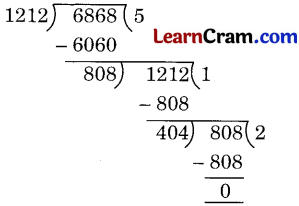HCF of 6868 and 1212 = 404
Now we have to find the HCF of 1111 and 404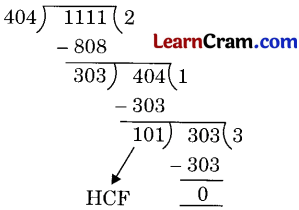Hence, the required HCF = 101.

(f) 1794, 2346, 4761
Solution:
Here, 4761 > 2346 > 1794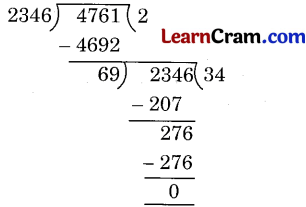HCF of 2346 and 4761 = 69
Now we have to find the HCF of 69 and 1794Hence, the required HCF = 69.(g) 70, 105, 175
Solution:
Here, 175 > 105 > 70HCF of 175 and 105 = 15
Now we have to find the HCF of 15 and 70.Hence, the required HCF = 5.

(h) 270, 450, 315
Solution:
Here, 450 > 315 > 270HCF of 450 and 315 = 45
Now we have to find the HCF of 45 and 270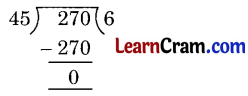Hence, the required HCF = 45.Question 4.
What is the HCF of:

(a) Two consecutive natural numbers
Solution:
1

(b) Two consecutive even numbers
Solution:
2

(c) Two consecutive odd numbers
Solution:
1

(d) Any two prime numbers
Solution:
1

Question 5.
Find the greatest number which divides 203 and 434 leaving remainder 5 in each case.
Solution:
We have to find the numbers which exactly divides
203 – 5 = 198 and
434 – 5 = 429
∴ We have to find the HCF of 198 and 429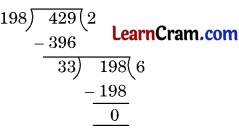Hence, the required number = 33.Question 6.
Find the greatest number which exactly divides 625 and 1433 leaving remainders 5 and 3 respectively.
Solution:
625 – 5 = 620 and
1433 – 3 = 1430
So we have to find HCF of 620 and 1430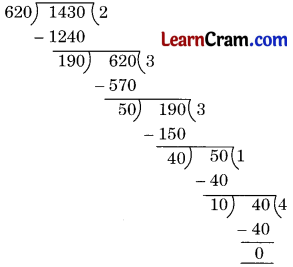Hence, the required number is 10.

Question 7.
The length, breadth and height of a room are 8.25 m, 6.75 m and 4.50 m respectively. Determine the longest tape which can measure the 3 dimensions of the rooms exactly.
Solution:
8.25 m = 8.25 × 100 cm = 825 cm
6.75 m = 6.75 × 100 cm = 675 cm
4.50 m = 4.50 × 100 cm = 450 cm
Now we have to find the HCF of 825, 675 and 450HCF of 825 and 675 = 75
Now we have to find the HCF of 75 and 450Hence, the HCF of the given number = 75
and the measurement of the tape = 75 cm or 0.75 m.Question 8.
There are 312 mango bites, 260 eclairs and 156 coffee bites in a box. These are to be put in packets so that each packet contains the same number of toffees. Find the maximum number of toffees in each packet.
Solution:
We have to find the HCF of 312, 260 and 156Now find the HCF of 52 and 156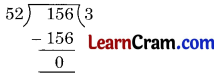Hence, the required number of toffees are 52.